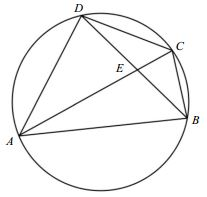# Cyclic Quadrilateral from the USAMO

### Xiaoxue Li Am Math Monthly, V 123, N 1, Jan 2016, p. 96

Here is problem 2, Part I, of the 28th United States of America Mathematical Olympiad.

Let $ABCD\;$ be a cyclic quadrilateral.Prove that

$|AB-CD+|AD-BC|\ge 2|AC-BD|.$

### Solution

It may be assumed without loss of generality that $AB\ge CD,\;$ $AD\ge BC,\;$ and $AC\ge BD.\;$ It then suffices to show that $(AB-CD)+(AD-BC)\ge 2(AC-BD).\;$ We introduce auxiliary points $A',\;$ $C',\;$ and $D'\;$ such that $EC-EC',\;$ $ED=ED',\;$ and $AA'=CD.\;$ Observe that triangles $CDE\;$ and $C'D'E\;$ are congruent.$\angle 1=\angle 3=\angle 2,\;$ implying that $C'D'\parallel AB.\;$ By the construction $\Delta EC'D'=\Delta ECD,\;$ in particular, $C'D'=CD=AA',\;$ making AD'C'A' a parallelogram. Thus, $AB-CD=A'B.\;$ Also

\begin{align} AC-BD &= AC-(a+b+BC')\\ &=AD'-BC'\\ &=A'C'-BC'\\ &\le A'B. \end{align}

It follows that $AB-CD\ge AC-BD.\;$ Similarly, $AD-BC\ge AC-BD.\;$ Adding these two inequalities yields the desired inequality. Equality holds when $ABCD\;$ is a rectangle.